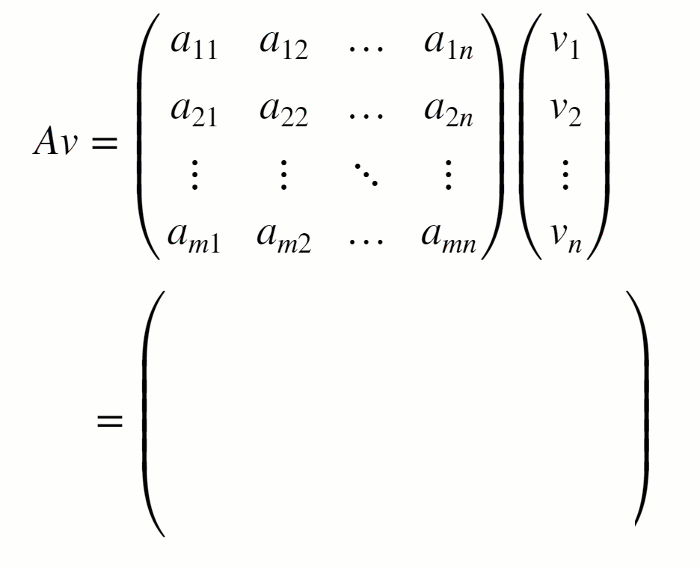## Matrix Methods of Linear Algebra

Version 1.0.4.0 (32.2 MB) by
Interactive courseware module that addresses fundamental matrix methods and linear systems taught in introductory linear algebra courses.

Updated 31 Jan 2023

From GitHub

# Matrix Methods of Linear Algebra

Curriculum Module
Created with R2020b. Compatible with R2020b and later releases.## Description

This curriculum module contains interactive live scripts that teach fundamental matrix methods commonly taught in introductory linear algebra courses. In the first part of each live script, students learn standard definitions, visualize concepts, and perform exercises on paper. Afterward, students practice complementary MATLAB® methods. These methods reinforce the discussed concepts and help students to develop an early familiarity with computational software. Each lesson concludes with an illustrative application. These live scripts can be used as part of a lecture, as activities in an instructional setting, or as an interactive assignment to be completed outside of class.

The instructions inside the live scripts will guide you through the exercises and activities. Get started with each live script by running it one section at a time. To stop running the script or a section midway (for example, when an animation is in progress), use the Stop button in the RUN section of the Live Editor tab in the MATLAB Toolstrip.

## Details

`matrixOperations.mlx, matrixOperationsSoln.mlx`
An interactive lesson that explores basic matrix operations.

Learning Goals:

• Define matrices and their basic arithmetic operations
• Calculate the result of matrix operations on paper and in MATLAB
• Explain the size requirements of matrix operations
• Compare symbolic and numeric matrix operations in MATLAB
• Apply matrix methods to modify grayscale images

`linearSystems.mlx, linearSystemsApplications.mlx, linearSystemsSoln.mlx, linearSystemsApplicationsSoln.mlx`
An interactive lesson that explores matrix representations of linear systems and their solutions.

Learning Goals:

• Write a linear system in matrix form
• Relate solutions of linear systems in 3-dimensions to their visualizations
• Solve systems of linear equations using row-reduction
• Solve systems of linear equations using matrix inverses
• Explain the solvability of a linear system in terms of the matrix determinant
• Use MATLAB to solve linear systems
• Apply matrix methods to linear regression and linear circuit analysis

`eigenanalysis.mlx, eigenanalysisApplications.mlx, eigenanalysisSoln.mlx, eigenanalysisApplicationsSoln.mlx`
An interactive lesson that explores eigenvectors, eigenvalues, and their applications.

Learning Goals:

• Visualize eigenvectors in 2-dimensions
• Solve for the eigenvalues and eigenvectors of a 2x2 matrix on paper and larger matrices using MATLAB
• Diagonalize 2x2 matrices on paper and larger matrices in MATLAB
• Explain linear system solvability in terms of eigenvalues
• Discuss defective matrices
• Use eigenanalysis to understand vibrations and the long term behavior of a Markov chain

## Products

MATLAB, Symbolic Math Toolbox™, Image Processing Toolbox™, Statistics and Machine Learning Toolbox™

The license for this module is available in the LICENSE.TXT file in this GitHub repository.

## Educator Resources

Have any questions or feedback? Contact the MathWorks online teaching team.

### Cite As

Ryan Weinstein (2023). Matrix Methods of Linear Algebra (https://github.com/MathWorks-Teaching-Resources/Matrix-Methods-of-Linear-Algebra/releases/tag/v1.0.4), GitHub. Retrieved .

##### MATLAB Release Compatibility
Created with R2020b
Compatible with R2020b and later releases
##### Platform Compatibility
Windows macOS Linux
##### Communities
More Files in the  Distance Learning Community

### Community Treasure Hunt

Find the treasures in MATLAB Central and discover how the community can help you!

Start Hunting!

#### SupportingFiles/MatrixOperations

Version Published Release Notes
1.0.4.0

See release notes for this release on GitHub: https://github.com/MathWorks-Teaching-Resources/Matrix-Methods-of-Linear-Algebra/releases/tag/v1.0.4

1.0.3

See release notes for this release on GitHub: https://github.com/MathWorks-Teaching-Resources/Matrix-Methods-of-Linear-Algebra/releases/tag/v1.0.3

1.0.2

See release notes for this release on GitHub: https://github.com/MathWorks-Teaching-Resources/Matrix-Methods-of-Linear-Algebra/releases/tag/v1.0.2

1.0.1

See release notes for this release on GitHub: https://github.com/MathWorks-Teaching-Resources/Matrix-Methods-of-Linear-Algebra/releases/tag/v1.0.1

1.0.0

To view or report issues in this GitHub add-on, visit the GitHub Repository.
To view or report issues in this GitHub add-on, visit the GitHub Repository.